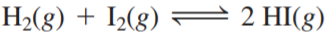×
Get Full Access to Chemistry: A Molecular Approach - 3 Edition - Chapter 14 - Problem 81e
Get Full Access to Chemistry: A Molecular Approach - 3 Edition - Chapter 14 - Problem 81e

×

# Consider the reaction: A reaction mixture at equilibriumISBN: 9780321809247 1

## Solution for problem 81E Chapter 14

Chemistry: A Molecular Approach | 3rd Edition

• Textbook Solutions
• 2901 Step-by-step solutions solved by professors and subject experts
• Get 24/7 help from StudySoup virtual teaching assistantsChemistry: A Molecular Approach | 3rd Edition

4 5 1 276 Reviews
30
2
Problem 81E

Consider the reaction:A reaction mixture at equilibrium at 175 K contains PH2 = 0.958 atm, PI2 = 0.877 atm, and PHI = 0.020 atm. A second reaction mixture, also at 175 K, contains PH2 = PI2 = 0.621 atm and PHI = 0.101 atm. Is the second reaction at equilibrium? If not, what will be the partial pressure of HI when the reaction reaches equilibrium at 175 K?

Step-by-Step Solution:

Solution 81EStep 1The given chemical reaction.The equilibrium constant expression for the above reaction is as follows.In terms to pressure.

Step 2 of 3

Step 3 of 3

##### ISBN: 9780321809247

The full step-by-step solution to problem: 81E from chapter: 14 was answered by , our top Chemistry solution expert on 02/22/17, 04:35PM. Chemistry: A Molecular Approach was written by and is associated to the ISBN: 9780321809247. Since the solution to 81E from 14 chapter was answered, more than 583 students have viewed the full step-by-step answer. The answer to “?Consider the reaction: A reaction mixture at equilibrium at 175 K contains PH2 = 0.958 atm, PI2 = 0.877 atm, and PHI = 0.020 atm. A second reaction mixture, also at 175 K, contains PH2 = PI2 = 0.621 atm and PHI = 0.101 atm. Is the second reaction at equilibrium? If not, what will be the partial pressure of HI when the reaction reaches equilibrium at 175 K?” is broken down into a number of easy to follow steps, and 69 words. This textbook survival guide was created for the textbook: Chemistry: A Molecular Approach, edition: 3. This full solution covers the following key subjects: reaction, atm, Equilibrium, contains, PHI. This expansive textbook survival guide covers 82 chapters, and 9454 solutions.

Unlock Textbook Solution